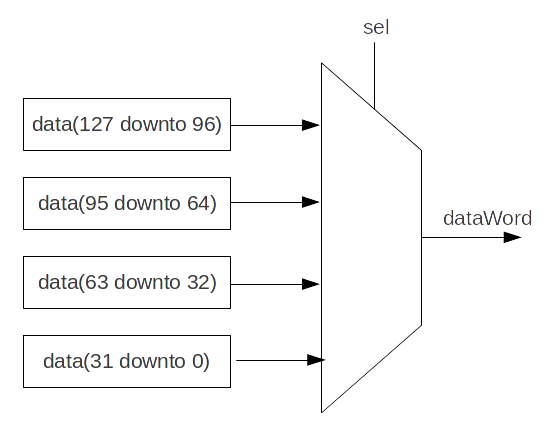You're reading an old version of this documentation.
For the latest stable release version, please have a look at master.

# When/Switch/Mux

## When

As in VHDL and Verilog, signals can be conditionally assigned when a specified condition is met:

```when(cond1) {
// Execute when cond1 is true
}.elsewhen(cond2) {
// Execute when (not cond1) and cond2
}.otherwise {
// Execute when (not cond1) and (not cond2)
}
```

## Switch

As in VHDL and Verilog, signals can be conditionally assigned when a signal has a defined value:

```switch(x) {
is(value1) {
// Execute when x === value1
}
is(value2) {
// Execute when x === value2
}
default {
// Execute if none of precedent conditions met
}
}
```

## Local declaration

It is possible to define new signals inside a when/switch statement:

```val x, y = UInt(4 bits)
val a, b = UInt(4 bits)

when(cond) {
val tmp = a + b
x := tmp
y := tmp + 1
} otherwise {
x := 0
y := 0
}
```

Note

SpinalHDL checks that signals defined inside a scope are only assigned inside that scope.

## Mux

If you just need a `Mux` with a `Bool` selection signal, there are two equivalent syntaxes:

Syntax

Return

Description

Mux(cond, whenTrue, whenFalse)

T

Return `whenTrue` when `cond` is True, `whenFalse` otherwise

cond ? whenTrue | whenFalse

T

Return `whenTrue` when `cond` is True, `whenFalse` otherwise

```val cond = Bool
val whenTrue, whenFalse = UInt(8 bits)
val muxOutput  = Mux(cond, whenTrue, whenFalse)
val muxOutput2 = cond ? whenTrue | whenFalse
```

## Bitwise selection

A bitwise selection looks like the VHDL `when` syntax.

### Example

```val bitwiseSelect = UInt(2 bits)
val bitwiseResult = bitwiseSelect.mux(
0 -> (io.src0 & io.src1),
1 -> (io.src0 | io.src1),
2 -> (io.src0 ^ io.src1),
default -> (io.src0)
)
```

Also, if all possible values are covered in your mux, you can omit the default value:

```val bitwiseSelect = UInt(2 bits)
val bitwiseResult = bitwiseSelect.mux(
0 -> (io.src0 & io.src1),
1 -> (io.src0 | io.src1),
2 -> (io.src0 ^ io.src1),
3 -> (io.src0)
)
```

`muxLists(...)` is another bitwise selection which takes a sequence of tuples as input. Below is an example of dividing a `Bits` of 128 bits into 32 bits:```val sel  = UInt(2 bits)
val data = Bits(128 bits)

// Dividing a wide Bits type into smaller chunks, using a mux:
val dataWord = sel.muxList(for (index <- 0 until 4) yield (index, data(index*32+32-1 downto index*32)))

// A shorter way to do the same thing:
val dataWord = data.subdivideIn(32 bits)(sel)
```